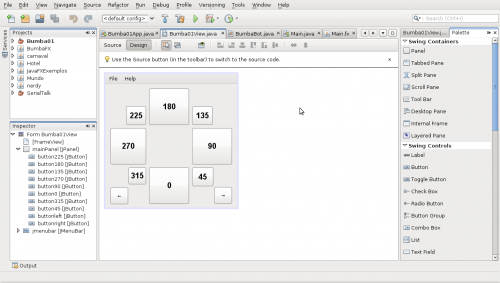# Tag: High-Current Darlington Transistor Arrays

I got a simple motor from a broken domestic printer. It’s a Mitsumi m355P-9T stepping motor. Any other common stepping motor should fits. You can find one in printers, multifunction machines, copy machines, FAX, and such.With a flexible cap of water bottle with a hole we make a connection between the motor axis and other objects.With super glue I attached to the cap a little handcraft clay ox statue.It’s a representation from a Brazilian folkloric character Boi Bumbá. In some traditional parties in Brazil, someone dress a structure-costume and dances in circular patterns interacting with the public.Controlling a stepper motor is not difficult.  There’s a good documentation on how to that on the Arduino Stepper Motor Tutorial. Basically it’s about sending a logical signal for each coil in a circular order (that is also called full step).Animation from rogercom.com.You’ll probably also use a driver chip ULN2003A or similar to give to the motor more current than your Arduino can provide and also for protecting it from a power comming back from the motor. It’s a very easy find this tiny chip on electronics or automotive  stores or also from broken printers where you probably found your stepped motor.```// Simple stepped motor spin // by Silveira Neto, 2009, under GPLv3 license // http://silveiraneto.net/2009/03/16/bumbabot-1/ int coil1 = 8; int coil2 = 9; int coil3 = 10; int coil4 = 11; int step = 0; int interval = 100;   void setup() { pinMode(coil1, OUTPUT); pinMode(coil2, OUTPUT); pinMode(coil3, OUTPUT); pinMode(coil4, OUTPUT); }   void loop() { digitalWrite(coil1, step==0?HIGH:LOW); digitalWrite(coil2, step==1?HIGH:LOW); digitalWrite(coil3, step==2?HIGH:LOW); digitalWrite(coil4, step==3?HIGH:LOW); delay(interval); step = (step+1)%4; }```

Writing a little bit more generally code we can create function to step forward and step backward.

My motor needs 48 steps to run a complete turn. So 360º/48 steps give us 7,5º per step. Arduino has a simple Stepper Motor Library but it doesn’t worked with me and it’s also oriented to steps and I’d need something oriented to angles instead. So I wrote some routines to do that.

For this first version of BumbaBot I mapped angles with letters to easy the communication between the programs.Notice that it’s not the final version and there’s still some bugs!

```// Stepped motor control by letters // by Silveira Neto, 2009, under GPLv3 license // http://silveiraneto.net/2009/03/16/bumbabot-1/   int coil1 = 8; int coil2 = 9; int coil3 = 10; int coil4 = 11;   int delayTime = 50; int steps = 48; int step_counter = 0;   void setup(){ pinMode(coil1, OUTPUT); pinMode(coil2, OUTPUT); pinMode(coil3, OUTPUT); pinMode(coil4, OUTPUT); Serial.begin(9600); }   // tells motor to move a certain angle void moveAngle(float angle){ int i; int howmanysteps = angle/stepAngle(); if(howmanysteps<0){ howmanysteps = - howmanysteps; } if(angle>0){ for(i = 0;i<howmanysteps; i++){ step(i%4); delay(delayTime); } }else{ for(i=0;i<howmanysteps;i++){ backstep(i%4); delay(delayTime); } } }   // tells motor to move to a certain angle void moveToAngle(float angle){ moveAngle(angle-actualAngle()); }   // actual stepper motor angle float actualAngle(){ return step_counter*stepAngle(); }   // angle made by each step float stepAngle(){ return 360.0/steps; }   // backward step void backstep(int coil){ digitalWrite(coil1, (coil==3)?HIGH:LOW); digitalWrite(coil2, (coil==2)?HIGH:LOW); digitalWrite(coil3, (coil==1)?HIGH:LOW); digitalWrite(coil4, (coil==0)?HIGH:LOW); step_counter--; }   // forward step void step(int coil){ digitalWrite(coil1, (coil==0)?HIGH:LOW); digitalWrite(coil2, (coil==1)?HIGH:LOW); digitalWrite(coil3, (coil==2)?HIGH:LOW); digitalWrite(coil4, (coil==3)?HIGH:LOW); step_counter++; }   void loop() { byte val; if(Serial.available()){ val = Serial.read(); switch (val) { case 'A': moveToAngle( 0); break; case 'B': moveToAngle( 45); break; case 'C': moveToAngle( 90); break; case 'D': moveToAngle(135); break; case 'E': moveToAngle(180); break; case 'F': moveToAngle(225); break; case 'G': moveToAngle(270); break; case 'H': moveToAngle(315); break; case 'I': backstep(1); backstep(0); break; case 'J': step(0); step(1); break; } Serial.print(val); } }```

In another post I wrote how create a Java program to talk with Arduino. We’ll use this to send messages to Arduino to it moves.[put final video here]

To be continued… :)